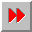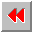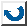EMA-XPS Online

EQUALP

```EQUALP
======

syntax: (equalp <object1> <object2>)
; Common Lisp
(is-nearly <object1> <object2> [<delta>])
; Babylon Lisp

This function compares both objects. It returns T,
when the objects are almost equal. EQUALP and
IS-NEARLY can not be compared for numbers: equalp
works like '='.

example:       >(equalp "a" "A")
T
>(equalp 1 1.01)
NIL

```EMA-XPS Online